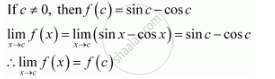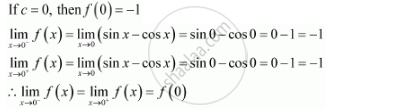Share

# Examine the Continuity of F, Where F is Defined by F(X) = {(Sin X - Cos X, If X != 0),(-1,If X = 0) - CBSE (Science) Class 12 - Mathematics

#### Question

Examine the continuity of f, where f is defined by

f(x) = {(sin x - cos x, if x != 0),(-1, "," if x = 0):}

#### Solution

The given function f is f(x) = {(sin x - cos x, if x != 0),(-1, "," if x = 0):}

It is evident that f is defined at all points of the real line.

Let c be a real number.

Case I:Therefore, f is continuous at all points x, such that x ≠ 0

Case II:Therefore, f is continuous at x = 0

From the above observations, it can be concluded that f is continuous at every point of the real line.

Thus, f is a continuous function.

Is there an error in this question or solution?

#### APPEARS IN

NCERT Solution for Mathematics Textbook for Class 12 (2018 to Current)
Chapter 5: Continuity and Differentiability
Q: 25 | Page no. 161

#### Video TutorialsVIEW ALL 

Solution Examine the Continuity of F, Where F is Defined by F(X) = {(Sin X - Cos X, If X != 0),(-1,If X = 0) Concept: Concept of Continuity.
S